# Practice Matrix Questions and Answers

## Matrix

Matrix forms an important part of the Non- Verbal Reasoning syllabus of various banking and competitive exams like SSC CGL, SSC CHSL, SBI PO, IBPS PO and many others.

### What is Matrix?

Matrix is a set of elements laid out in tabular form (in rows and columns).

Matrix coding is a method to represent the numbers/letters/figures, where one digit/number/figure will be missing. The numbers/digits/figures will follow a particular pattern/logic.

The candidates are required to decipher that logic/pattern to solve the given question.

### Types of Matrix Questions

The matrix questions can be based on the following types-

### Missing Number/Alphabet

Here, the characters can be a set of numbers/alphabets or a combination of numbers and letters that follow a certain pattern. The candidates are required to figure out the logic behind the pattern and answer the questions based on the options.

For example-

Q1)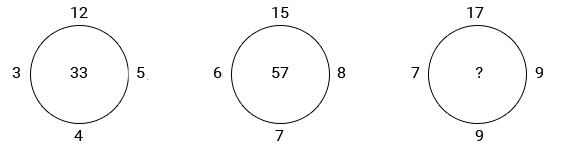• 80
• 90
• 72
• 64

Solution: Option b

Explanation:

The pattern is: (12×4)-(5×3)=33,(15×7)-(8×6)=57.

∴ Missing number =(17×9)-(9×7)=90.

#### Common Patterns for Missing Numbers/Alphabets Questions

• It could be a sum of two numbers divided by a consonant
• It could be average of numbers
• It could be in the form of alphabets, wherein the alphabets are increased by consonant or increased by square of numbers or increased by prime numbers.
• The difference between numbers could be in the pattern of squares or cubes of numbers.
• The difference could be the sum of adjacent numbers.

### Figure Matrix

In such types of questions, more than one set of figures is given in the form of a matrix. All the figures follow the same rule. The candidates are required to analyze the complete sets and find out the common pattern to complete the incomplete figure.

For example-

Q1) Problem Figure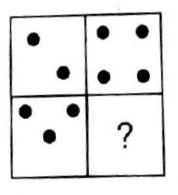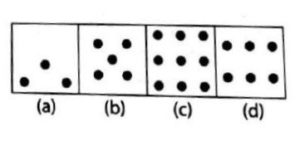• A
• B
• C
• D

Solution: option D

Explanation:

In the upper row, the number of dots in the second is twice the number of dots in the first figure. Similarly, in the lower row, the number of dots in the second figure must be twice the number of dots in the lower first figure. So, the number of dots in the missing segment is = 2×3 = 6. Thus the correct answer is D. (d).

Q1)

###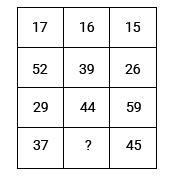• 41
• 43
• 31
• 40

Solution: Option 1

Explanation:

In each row the middle term is average of extreme terms 17+15/2 = 16, 52+26/2 = 39. So, missing number =37+45/2 = 41.Hence, the answer is option 1

Q2)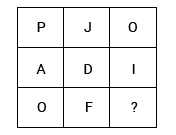• F
• G
• H
• K

Solution: Option 1

Explanation:

Positions of alphabets (A to Z) is (1-26)

Thus, we have:

In the first column, P-A=16-1=15=O.

In the second column, J-D=10-4=6=F.

So, in the third column, missing letter =O-I=15-9=6 =F

So the answer is option 1

Q3) Problem figure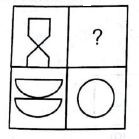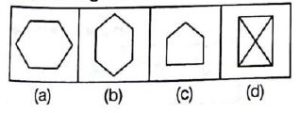• A
• B
• C
• D

Solution: Option B

Explanation:

The two parts of the first figure are rearranged and joined along the longer sides. The common side is then removed to form the second figure. Hence the answer is B.

Q4) Problem figure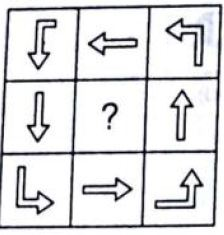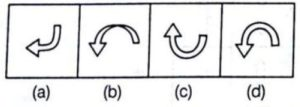• A
• B
• C
• D

Solution: Option D

Explanation:

In the question figure, all the arrows are headed in the same direction. They are all pointing in an anticlockwise direction. So the answer can either be (b) or (d). In (b), the shape of the arrow is unnecessarily peculiar. Hence the only shape that fits the matrix will be D.

Q5)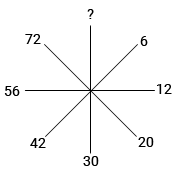• 90
• 76
• 84
• 88

Solution: Option 1

Explanation:

Going clockwise starting with 6 pattern is 6=22+2, 12 = 32+3,

20 =42+4, 30 =52+5, 42 =62+6

56 = 72+7, 72=82+8, so missing number is 92+9=90 , So answer is option 1

### Conclusion

Matrix can be a challenging topic for some candidates if their basic concepts are not clear. This is because this topic tests the visual as well as analytical skills of the candidates. You need to be able to figure out the logic/reasoning behind the pattern in the given question. However, you can master this topic by practicing as many questions as possible. Candidates can expect around 4 to 5 questions from this topic.

×
×